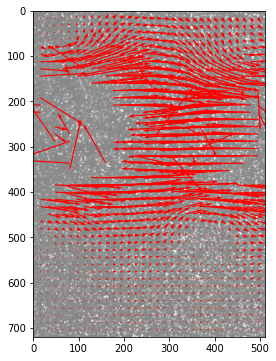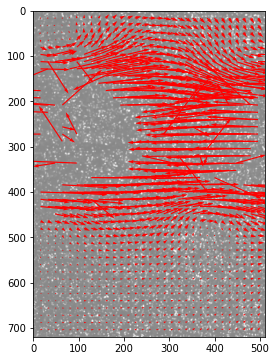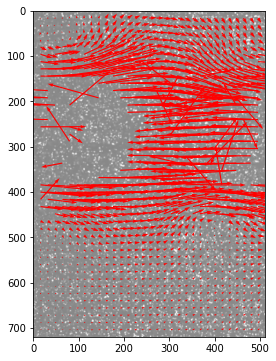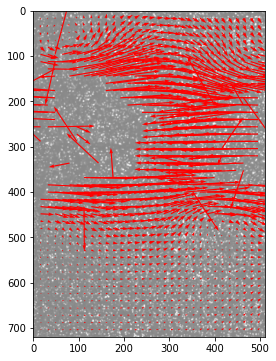In :
# we need to read frames from the movie
# so we install opencv-python - change the next cell type to "Code"

# !pip install opencv-python
In :
import cv2

In :
import numpy as np
import matplotlib.pyplot as plt

In :
from openpiv import pyprocess, piv

In :
# the video is the jet PIV from Youtube
# https://www.youtube.com/watch?v=EeS1rYMZUxI&ab_channel=USUExperimentalFluidDynamicsLab
# all the rights reserved to the authors

vidcap = cv2.VideoCapture('../test_movie/videoplayback.mp4')
success, image1 = vidcap.read()
count = 0
U = []
V = []

while success and count < 10:
# cv2.imwrite("frame%d.jpg" % count, image)     # save frame as JPEG file
success, image2 = vidcap.read()
# print('Read a new frame: ', success)
if success:
x,y,u,v = piv.simple_piv(image1.sum(axis=2), image2.sum(axis=2),plot=True);
image1 = image2.copy()
count += 1
U.append(u)
V.append(v)